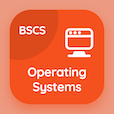Management Online Courses (MBA)

MBA Statistics Quizzes

MBA Statistics Quiz PDF - Complete

# T-Test Multiple Choice Questions p. 23

Study T-Test multiple choice questions and answers, t-test quiz answers PDF 23 to study MBA Statistics course online. Hypothesis Testing MCQ trivia questions, t-test Multiple Choice Questions (MCQ) for online college degrees. "T-Test MCQ" PDF eBook: ordinary least square assumptions, data elements, variables and observations, multicollinearity, estimated multiple regression equation, t-test test prep for cheapest MBA programs.

"In t-test, if the calculated value is greater than the critical value in absolute form, then" MCQ PDF: do not reject null hypothesis, reject null hypothesis, do nothing, and estimate again for school for business online. Learn hypothesis testing questions and answers to improve problem solving skills .

## T-Test Questions and Answers MCQs

MCQ: In t-test, if the calculated value is greater than the critical value in absolute form, then

Reject Null hypothesis
Do not reject null hypothesis
Do nothing
Estimate again

MCQ: In the lin-log model equation, computed elasticity is equals to

β1 *(1/y)
β2 * (1/y)
β1 *y
β2 *y

MCQ: As a rule of thumb, if the value of VIF exceeds 10 and r² is greater than 0.901, then there's

Biased factoring
Homogeneity
Multicollinearity
Unbiased factoring

MCQ: Average value of any data set is known to be its

Mean
Mode
Standard deviation
Standard Error from Origin

MCQ: The explanatory values in a given sample of OLS methods are assumed to be

Same
Fixed term
Not same
Relative

### Practice Quizzes from Business Statistics Course